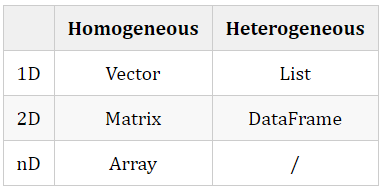# 东哥和刘亦菲的故事# 1. 问题

R 语言作为统计学家御用语言，数据类型和语法规则和常见的给程序员使用的编程语言多有不同。不能说人家坏，只是不适合。

# 2. 分析## 2.1. Vector

Vector 是 R 里面最基础的数据类型。简单的话可以理解成一组同质数据组成的数组。 Vector 可以用c函数创建。c代表 combine，可以把多个数据合并成一个数据。例如

> avec = c(1, 2, 3) 1 2 3

Vector 可以进行集合运算。比如

> b = a+100 101 102 103> b + a 102 104 106

## 2.2. Factor

Factor 可以看做 Integer Vector 加上 Label。比如

> f = factor(c('a', 'b', 'c', 'a'))> f a b c aLevels: a b c> str(f) Factor w/ 3 levels "a","b","c": 1 2 3 1

## 2.3. matrix

matrix 是单一类型组成的二维变量，比如

> mat = matrix(c('a', 'b', 1, 2), nrow=2)> mat     [,1] [,2][1,] "a"  "1" [2,] "b"  "2" 

## 2.4. List

List 可以包含多种数据类型，来者不拒，摘到篮子里的都是菜。比如

> list1 = list(a = a, b= b, f = f)> list1$a "101" "102" "103" "python"$b 101 102 103$f a b c aLevels: a b c> list2 = list(a, b, f)> list2[] "101" "102" "103" "python"[] 101 102 103[] a b c aLevels: a b c 复制代码 可见，指定命名就可以用$访问，否则就只能用[[]]访问。

## 2.5. DataFrame

DataFrame 不用多说，R 里面最常用的数据类型。可以简单的理解为 Excel 表格的数据库，各列可以有不同类型的数据，但列内类型必须一致。

> df = data.frame(a, b, f)> df       a   b f1    101 102 a2    102 103 b3    103 104 c4 python 105 a> class(df) "data.frame"> class(df$a) "factor"> class(df$b) "numeric"> class(df\$f) "factor"

# 3. 转换

vector 性格单纯，可做多样使用。最常见的是转换成 DataFrame，比如

> dfv = data.frame(unl)> dfv     unl1    1012    1023    1034 python5    1016    1027    1038      1> dav = as.data.frame(unl)> dav     unl1    1012    1023    1034 python5    1016    1027    1038      1> dav == dfv      unl[1,] TRUE[2,] TRUE[3,] TRUE[4,] TRUE[5,] TRUE[6,] TRUE[7,] TRUE[8,] TRUE

# 5. 交流Lily

# 6. 扩展

## 6.1. 参考文献

1. Dr. P. Prakash 和 A. S. K. Rao, R Data Structures and Algorithms. 2016.

2. H. Wickham 和 G. Grolemund, R for data science: import, tidy, transform, visualize, and model data, First edition. Sebastopol, CA: O’Reilly, 2016.Data2Science## 评论 (1 条评论)2020 年 05 月 19 日 16:58